# Quiz 13: Queuing Analysis

Queueing system is a system that has any type of waiting lines(queues). Various models can be built from the queueing system to calculate the parameters like wait time, length of the queue, etc. Poisson process is a counting process in which events occur on a continuous basis with mean rate ?. It is represented as { X(t); t = 0}. a. In a single-server waiting line model, calculate the average waiting time in the queue using the following formula:Since a victim arrives after every 7 minutes, calculate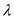as follows:Since a victim is serviced after every 4.5 minutes, calculate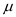as follows:Calculate the waiting time as follows:Thus, the average waiting time for victims will be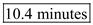. Now, when the distribution of service times is undefined,= 9 as aforementioned and calculateas follows:Compute the average time using the following formula: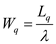Here,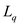= expected number of victims in the queue Now, computeusing the following formula: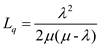Obtain the values of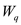andas follows:Thus, the average waiting time for victims when the distribution of service times is undefined will be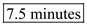. b. Here, a multi-server model is considered with= 9 as aforementioned and calculateas follows:Calculate the expected number of customers in the system using the following formula: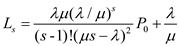Here, compute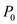using the following formula:Here,Calculateand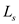as shown below:Calculate the average waiting time for a victim in the system as follows:Calculate the average waiting time for a victim in the queue as follows:c. Here,= 18 (number of victims) Now, calculate the average waiting time for a victim in the system as follows:Calculate the average number of victims in the queue as follows:Calculate the average waiting time for a victim in the queue as follows:d. Here, s = 2 (number of servers) Now, calculateandas follows:Calculateandas shown below:Now, calculate the average waiting time for a victim in the system as follows:Calculate the average number of victims in the queue as follows:Calculate the average waiting time for a victim in the queue as follows:e. This is a multiple server model with s = 5 (number of physicians). Since a victim arrives after every 8.5 minutes, calculateas follows:Calculateas follows:Now, computeandas shown below:Calculate the average waiting time for a victim in the system as follows:Calculate the average waiting time for a victim in the queue as follows:f. Here,= 23 (number of victims) Now, calculate the average waiting time for a victim in the system as follows:Calculate the average number of victims in the queue as follows:Calculate the average waiting time for a victim in the queue as follows:g. The most useful model in analyzing the disaster situation will be model e (multi-server model with five physicians in hospital M) since it has the least waiting time for the victims in the queue and the system. Also, the length of the waiting line is the least in the model. To use other models together to analyze the disaster situation , the pros and cons of each model needs to be adjusted so that the overall waiting time and the length of the waiting queue is minimum. This can be done by incorporating more physicians in model b or more emergency vehicles in model c. Other type of waiting line models that might be useful in analyzing the disaster situation will be as follows: • Jockeying - This means the movement between the queues is possible which occurs when there are more than one servers which in turn is preceded by queue. • The service time of the victim is not exponentially distributed or undefined. • Service is provided to the victims not in first-come first basis but on the basis of alphabetical order. • There can also be a model in which victims can be shifted to another hospital if the queue is very long in the hospital to which the patient is first taken to.
BEI airport has a problem in staffing and security checking. In the peak season it is having the problem of long waiting lines and waiting times. BEI decided to forecast the airport passenger arrival in order to find out the number of check points that are needed to avoid the rush and waiting time for 2 hour time segment. Service times are exponentially distributed with a mean of 11.6 seconds. The operational characteristics for the above problem can be calculated using the single server model. The probability that no customers are in the queuing system (either in the queue or being served)Calculation of the security check points for two hour time segment- By applying the above formulas we can now calculate the values of Po, Pw, L, Lq, W and Wq. • 4:00 A.M to 6:00 A.MThe number of check points required is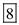. • 6:00 A.M to 8:00 A.MThe number of check points required is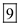. • 8:00 A.M to 10:00 AMThe number of check points required is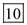• 10:00 A.M to NOONThe number of check points required is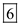• NOON- 2:00 PMThe number of check points required is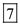• 2:00 P.M TO 4:00 P.MThe number of check points required is• 4:00 P.M TO 6:00 P.M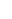The number of check points required is. • 6:00 P.M to 8:00 P.MThe number of check points required is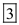• 8:00 P.M to 10:00 P.MThe number of check points required is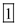. The number of security checkpoints is sufficient to keep passengers move freely through the security systems without excessive delays.
Determine the order service configuration to achieve their set goals of meeting the targets in the form of Excel Spreadsheet. As per the stated case, the current system of achieving the company goals is not feasible and the management of NB does not think if they work 8hours, 5 days per week, they would be able to serve the customers effectively. Calculate the second alternative that is 7 days and 8 hours service in the Excel spreadsheet. The exhibit shows the average time and number taken in the queue and the system. Besides that, the spreadsheet throws light on the total cost that was incurred in meeting the calls.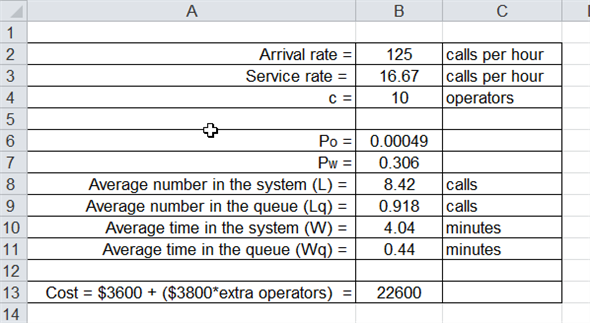Insert the formulas in the following way: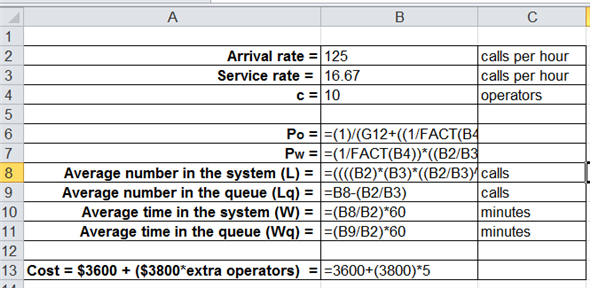The terms for 9 days service are also shown below: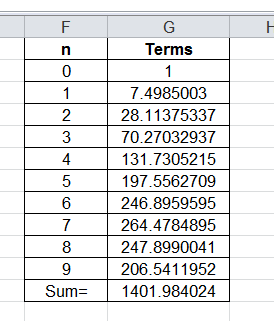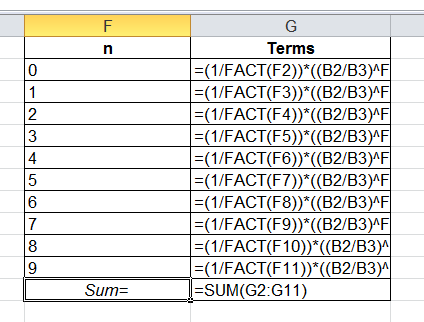Calculate the third alternative that is 5 days and 16 hours service in the Excel spreadsheet. The exhibit shows the average time and number taken in the queue and the system. Besides that, the spreadsheet throws light on the total cost that was incurred in meeting the calls.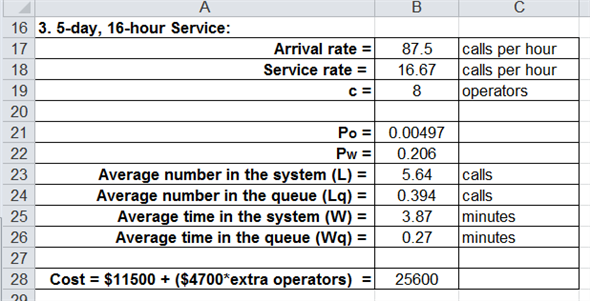Insert the formulas in the following way: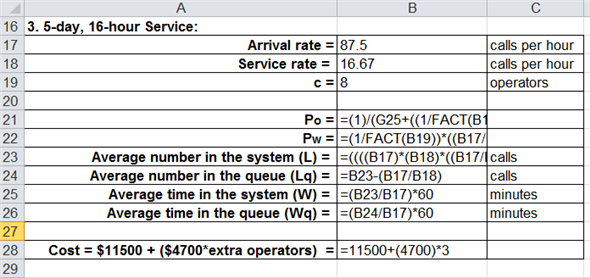The terms for 7 days service are also shown below: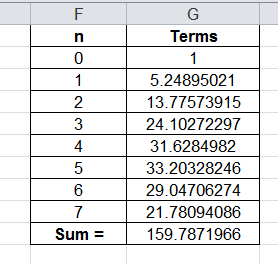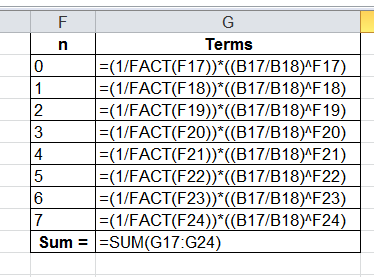Calculate the fourth alternative that is 7 days and 16 hours service in the Excel spreadsheet. The exhibit shows the average time and number taken in the queue and the system. Besides that, the spreadsheet throws light on the total cost that was incurred in meeting the calls.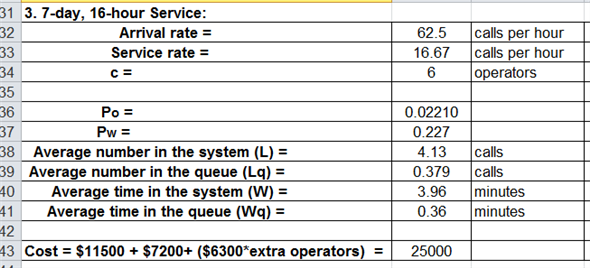The terms for 5 days service are also shown below: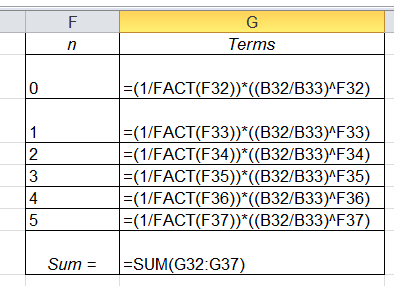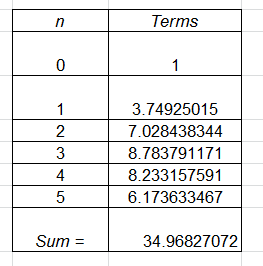Among all the alternatives, second alternative would be the most feasible option for the company P. The cost incurred in meeting the targets is the least that is \$22,600 among all the other alternatives.